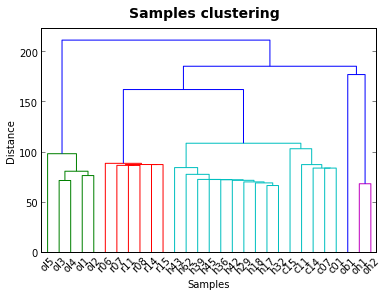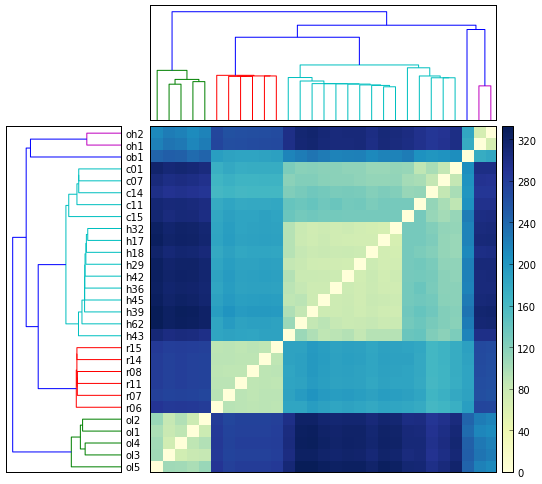# Comparison of Python packages for hierarchical clustering¶

Example data has been downloaded from the open access Human Gene Expression Atlas and represents typical data bioinformaticians work with.

It is "Transcription profiling by array of brain in humans, chimpanzees and macaques, and brain, heart, kidney and liver in orangutans" experiment in a tab-separated format.

In :
data = np.genfromtxt("data/ExpRawData-E-TABM-84-A-AFFY-44.tab",names=True,usecols=tuple(range(1,30)),dtype=float, delimiter="\t")

In :
print len(data)
print len(data.dtype.names)

54674
29

In :
data_array = data.view((np.float, len(data.dtype.names)))
data_array = data_array.transpose()


Let's have a look at how our data actually looks like

In :
print data_array

[[  6.3739767   5.986182    7.468118  ...,  11.745089   13.277803
13.067169 ]
[  6.4981704   4.861167    6.9479957 ...,  11.33983    13.031992
12.7244425]
[  6.271771    5.5666986   6.9435835 ...,  11.840397   13.332481
13.051369 ]
...,
[  6.112102    5.0324726   6.93343   ...,  11.411251   13.084589
12.768577 ]
[  6.359853    6.12955     8.460821  ...,  10.814936   12.888793
12.673793 ]
[  6.010906    5.4538217   6.8208113 ...,  11.467866   13.08952    12.792053 ]]


## 1. Samples clustering using scipy¶

First, we'll implement the clustering using scipy modules

In :
import numpy as np
import matplotlib.pyplot as plt
from scipy.spatial.distance import pdist, squareform

In :
data_dist = pdist(data_array) # computing the distance

In :
dendrogram(data_link,labels=data.dtype.names)
plt.xlabel('Samples')
plt.ylabel('Distance')
plt.suptitle('Samples clustering', fontweight='bold', fontsize=14);Plotting a heatmap that many R users are used to is a tricky endevour in Python

In :
# Compute and plot first dendrogram.
fig = plt.figure(figsize=(8,8))
# x ywidth height
Z1 = dendrogram(Y, orientation='right',labels=data.dtype.names) # adding/removing the axes
ax1.set_xticks([])

# Compute and plot second dendrogram.
Z2 = dendrogram(Y)
ax2.set_xticks([])
ax2.set_yticks([])

#Compute and plot the heatmap
idx1 = Z1['leaves']
idx2 = Z2['leaves']
D = squareform(data_dist)
D = D[idx1,:]
D = D[:,idx2]
im = axmatrix.matshow(D, aspect='auto', origin='lower', cmap=plt.cm.YlGnBu)
axmatrix.set_xticks([])
axmatrix.set_yticks([])

# Plot colorbar.
plt.colorbar(im, cax=axcolor)

Out:
<matplotlib.colorbar.Colorbar instance at 0x109a023f8>## 2. Fastcluster implementation is superior in memory usage¶

In :
from fastcluster import *
plt.xlabel('Samples')
plt.ylabel('Distance')
plt.suptitle('Samples clustering', fontweight='bold', fontsize=14);
show()

10 loops, best of 3: 41.9 ms per loop## 3. BioPython. Unique data exploration posibilities.¶

Biopython example would be rather specfic to bioinformatics. The output of the clustering should be viewed with TreeView program First, we need to read the data to a special bioipython.record object.

In [ ]:
from Bio.Cluster import *
handle = open("../data/ExpRawData-E-TABM-84-A-AFFY-44.tab")

genetree = record.treecluster(method='s')